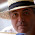## 10 August 2014

### Quiz 3: Take input till user presses "n".

Problem:
Keep taking input from user till user presses "n" and sum all inputs.

Sample Input:
Taken as <STDIN>

Sample Output:
Sum is xyz

Solution:

my \$tmp = 1;
my \$sum = 0;
my \$var = 0;
print "press n when you want to stop entering numbers\n";
do{
print "Enter number \$tmp\n";
\$var = <STDIN>   ;
\$sum = \$sum+\$var;
++\$tmp;
}  until(\$var == "n") ;
print "sum is \$sum";

Tips:
• Use ==, when comparing

1.You want:

until (\$var eq "n");

You also want to chomp() the input.

1.@Dave: it works perfectly

2.#!/usr/bin/perl -w
use strict;

print "print enter 1st digit:";
my \$string = ;

my @input = qw();
my \$sum = 0;
push(@input,\$string);
do
{
print "print enter digit:";
\$string = ;
chomp(\$string);
push(@input,\$string);
}until(\$string == "n");

chomp(@input);
print "@input\n";

for(my \$i=0;\$i<\$#input;\$i++)
{
\$sum = \$sum+\$input[\$i];
}
print "\$sum";

1.Correction 1: use \$string eq "n" instead of ==
Correction 2: wont work when user press n directly ie when sum is 0